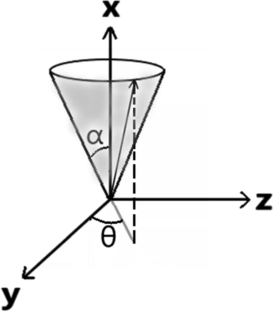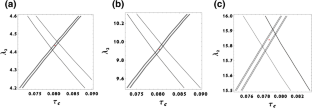# Relaxation in Pulsed EPR: Thermal Fluctuation of Spin-Hamiltonian Parameters of an Electron-Nuclear Spin-Coupled System in a Malonic Acid Single Crystal in a Strong Harmonic-Oscillator Restoring Potential

## Abstract

Random fluctuations in the $$\tilde{g}$$ and $$\tilde{A}$$ matrices of a spin system due to thermal motion of a molecule are specifically considered to calculate the relaxation matrix for the four-level electron-nuclear spin-coupled system ($$S = 1/2$$; $$I = 1/2$$) in a malonic acid crystal, using the formalism outlined by Lee et al. (J Chem Phys 98:3665–3689, 1993). The correlation time, τc, and the value of the parameter λ, characterizing the harmonic-oscillator restoring potential of the small-amplitude fluctuation of the director of the malonic-acid molecule due to thermal motion are estimated from the knowledge of the experimental values of (τc, λ). The four electronic, $$T_{2e} ,$$ and the two nuclear, $$T_{{2{\text{n}}}}$$, spin relaxation times are calculated to be functions of (τc, λ) governing the fluctuations. The values of (τc, λ), evaluated with these expressions, when fitted to the experimental values of $$T_{2e}$$ and $$T_{2n}$$, assuming the molecule to be in the ground state (n = 0) in the harmonic-oscillator potential, a rather narrow region of (τc, λ) values about τc = 0.081 μs and $$\lambda = 4.4$$ is found. These values are then used to calculate the time-dependent echo-ELDOR signal by the relevant Liouville von-Neumann (LVN) equation. The resulting Fourier transform is found to be in excellent agreement with the experimental data. The (τc, λ) values for the excited states described by $$n = 1,2$$ have also been calculated, although these states are unlikely to be populated at room temperature.

This is a preview of subscription content, access via your institution.Fig. 1Fig. 2Fig. 3

## Data Availability

The data that support the findings of this study are available from the corresponding author, SKM, upon reasonable request.

## Code Availability

The Matlab code to calculate the echo-ELDOR signal is published in  by the authors of this paper. It suffices to replace the relaxation matrix in that code by the relaxation matrix calculated in the present work to get Fig. 3.

## References

1. 1.

S. Lee, B.R. Patyal, J.H. Freed, J. Chem. Phys. 98, 3665–3689 (1993)

2. 2.

D. Gamliel, J.H. Freed, J. Magn. Reson. 89, 60–93 (1990)

3. 3.

C.P. Slichter, Principles of Magnetic Resonance, vol. 1 (Springer Verlag, New York, 1989)

4. 4.

S.A. Dzuba, E.S. Salnikov, L.V. Kulik, Appl. Magn. Reson. 30, 637 (2006)

5. 5.

S.K. Misra, H.R. Salahi, Magn. Reson. Solids 22(1), 20101 (2020)

6. 6.

C.F. Polnaszek, G.V. Bruno, J.H. Freed, J. Chem. Phys. 58(8), 3185–3199 (1973)

7. 7.

L. Kevan, R.N. Schwartz, Time Domain Electron Spin Resonance (Wiley, New York, 1979), Chap. 3, pp. 80–84

8. 8.

S.K. Misra (ed.), Multifrequency Electron Paramagnetic Resonance: Theory and Applications (Wiley-VCH, Weinheim, 2011), Chap. 10, pp. 469–471

9. 9.

S.K. Misra, H.R. Salahi, J. Appl. Theor. Phys. Res. 3(2), 9–48 (2019)

10. 10.

S.K. Misra, H.R. Salahi, L. Li, Magn. Reson. Solids 21, 19505 (2019)

11. 11.

D. Gamliel, H. Levanon, Stochastic Processes in Magnetic Resonance (World Scientific, Singapore, 1995)

12. 12.

J. Jeener, Adv. Magn. Reson. 10, 1–51 (1982)

13. 13.

A.G. Redfield, IBM J. Res. Dev. 1(1), 19–31 (1957)

14. 14.

A. Abragam, The Principles of Nuclear Magnetism (Oxford University Press, Oxford, 1961)

15. 15.

S.K. Misra, P.P. Borbat, J.H. Freed, Appl. Magn. Reson. 36, 237–258 (2009)

Download references

## Acknowledgements

We are grateful to the Natural Sciences and Engineering Research Council of Canada and Bridge funding from Concordia University, for financial support. Substantive helpful discussions on the model used with Professor Freed of Cornell University, USA, are gratefully acknowledged. Thanks are due to Dr. Lin Li for helpful discussions and a preliminary version of the source code.

## Author information

Authors

### Corresponding author

Correspondence to Sushil K. Misra.

## Additional information

### Publisher's Note

Springer Nature remains neutral with regard to jurisdictional claims in published maps and institutional affiliations.

## Appendices

### Appendix A: Spin Hamiltonian

For the specific case of a single nucleus ($$I = 1/2$$) interacting with an unpaired electron ($$S = 1/2$$) by hyperfine (HF) interaction, where the HF matrix has the same principal axes as those of the g matrix, the total Hamiltonian is

$$\hat{H} = \hat{H}_{0} + \hat{H}_{1} ,$$
(19)

where

$$\hat{H}_{0} = CS_{z} - \omega_{n} I_{z} + AS_{z} I_{z} + \frac{1}{2}BS_{z} I_{ + } + \frac{1}{2}B^{*} S_{z} I_{ - } .$$
(20)

In Eq. (20) the coefficients are expressed as follows:

$$\begin{gathered} C = \frac{{\beta_{e} B_{0} }}{h}\left[ {\overline{g} + F\frac{1}{2}\left( {3\cos^{2} \beta - 1} \right) + F^{\left( 2 \right)} \sin^{2} \beta \cos \left( {2\gamma } \right)} \right] \hfill \\ A = - 2\pi \left[ {\overline{a} + D\frac{1}{2}\left( {3\cos^{2} \beta - 1} \right) + D^{\left( 2 \right)} \sin^{2} \beta \cos \left( {2\gamma } \right)} \right] \hfill \\ B = - 4\pi \left\{ {D\frac{3}{4}\sin \beta \cos \beta - D^{\left( 2 \right)} \frac{1}{2}\sin \beta \left[ {\cos \beta \cos \left( {2\gamma } \right) - i\sin \left( {2\gamma } \right)} \right]} \right\}, \hfill \\ \end{gathered}$$
(21)

where

$$\begin{gathered} \overline{g} = \frac{1}{3}\left( {g_{xx} + g_{yy} + g_{zz} } \right);\; \overline{a} = \frac{1}{3}\left( {A_{xx} + A_{yy} + A_{zz} } \right); F = \frac{2}{3}\left( {g_{zz} - \frac{1}{2}\left( {g_{xx} + g_{yy} } \right)} \right); \hfill \\ D = \frac{2}{3}\left( {A_{zz} - \frac{1}{2}\left( {A_{xx} + A_{yy} } \right)} \right);\; F^{\left( 2 \right)} = \frac{1}{2}\left( {g_{xx} - g_{yy} } \right); D^{\left( 2 \right)} = \frac{1}{2}\left( {A_{xx} - A_{yy} } \right). \hfill \\ \end{gathered}$$
(22)

Here $${\Omega }\left( {\eta ,\beta ,\gamma } \right) = \left( {0, - {\Theta },0} \right)$$ are the Euler angles, which describe the orientation of the $$\tilde{g}$$ and $$\tilde{A}$$ matrices with respect to the static magnetic field, with $$\Theta$$ being the angle between the z axis of the magnetic frame and the Z-axis of the laboratory fixed frame.

The eigenvalues of the Hamiltonian, $$\hat{H}_{0}$$, as given by Eq. (20) are 

$$E_{a} = \frac{C}{2} + \frac{1}{2}\omega_{\alpha } ;\;E_{b} = \frac{C}{2} - \frac{1}{2}\omega_{\alpha } ;\;E_{c} = - \frac{C}{2} - \frac{1}{2}\omega_{\beta } ;\;E_{d} = - \frac{C}{2} + \frac{1}{2}\omega_{\beta } ,$$
(23)

where $$\omega_{\alpha }$$, $$\omega_{\beta }$$ are

$$\omega_{\alpha } = \left[ {\left( {\omega_{n} - \frac{A}{2}} \right)^{2} + \left( \frac{B}{2} \right)^{2} } \right]^{1/2} ;\;\omega_{\beta } = \left[ {\left( {\omega_{n} + \frac{A}{2}} \right)^{2} + \left( \frac{B}{2} \right)^{2} } \right]^{1/2} .$$
(24)

The eigenvectors are

$$\begin{gathered} \left| a \right. = \left[ {\begin{array}{*{20}c} {c_{1} } & { - c_{2} } & 0 & 0 \\ \end{array} } \right]^{{\text{T}}} ; |b = \left[ {\begin{array}{*{20}c} {c_{2} } & {c_{1} } & 0 & 0 \\ \end{array} } \right]^{{\text{T}}} \hfill \\ \left| c \right. = \left[ {\begin{array}{*{20}c} 0 & 0 & {c_{3} } & { - c_{4} } \\ \end{array} } \right]^{{\text{T}}} ; \left| d \right. = \left[ {\begin{array}{*{20}c} 0 & 0 & {c_{4} } & {c_{3} } \\ \end{array} } \right]^{{\text{T}}} , \hfill \\ \end{gathered}$$
(25)

where the superscript T denotes the transpose. The coefficient $$c_{i} , i = 1, \ldots ,4$$, in the above expressions are

$$\begin{gathered} c_{1} = \frac{1}{\sqrt 2 }\left[ {1 \pm \frac{{\left( {A/2} \right) - \omega_{n} }}{{\omega_{\alpha } }}} \right]^{1/2} ;c_{2} = - \frac{1}{\sqrt 2 }\left[ {1 \mp \frac{{\left( {A/2} \right) - \omega_{n} }}{{\omega_{\alpha } }}} \right]^{1/2} ; \hfill \\ c_{3} = \frac{1}{\sqrt 2 }\left[ {1 + \frac{{\left( {A/2} \right) + \omega_{n} }}{{\omega_{\beta } }}} \right]^{1/2} ;c_{4} = - \frac{1}{\sqrt 2 }\left[ {1 - \frac{{\left( {A/2} \right) + \omega_{n} }}{{\omega_{\beta } }}} \right]^{1/2} . \hfill \\ \end{gathered}$$
(26)

Here $$\omega_{n}$$ is the nuclear Larmor frequency.

### Appendix B: Solution of Liouville von Neumann (LVN) equation

The equation of motion of the reduced density matrix, $$\chi \left( t \right) = \rho \left( t \right) - \rho 0$$, applicable during free evolution, i.e. in the absence of any time-dependent part in the Hamiltonian, is expressed as Liouville von Neumann (LVN) equation in Liouville space as follows [9,10,11,12,13,14,15].

$$\frac{{{\text{d}}\widehat{{\hat{\chi }}}}}{{{\text{d}}t}} = - i\widehat{{\hat{L}}}\widehat{{\hat{\chi }}} - \widehat{{\hat{R}}}\widehat{{\hat{\chi }}} = - \widehat{{\hat{L}}}^{\prime}\widehat{{\hat{\chi }}},$$
(27)

where $$\widehat{{\hat{\chi }}}$$ is the column vector in Liouville space corresponding to $$\chi \left( t \right)$$ in Hilbert space as defined above, $$\widehat{{\hat{R}}}$$ is the relaxation superoperator and $$\widehat{{\hat{L}}}^{\prime}$$ is the Liouvillian, which is defined as

$$\widehat{{\hat{L}}}^{^{\prime}} \equiv i\left[ {I_{4} \otimes \hat{H}_{0} - \left( {\hat{H}_{0} } \right)^{T} \otimes I_{4} } \right] + \widehat{{\hat{R}}},$$
(28)

with $$\hat{H}_{0}$$ being the spin Hamiltonian in Hilbert space and $$I_{n}$$ is a 4 $$\times$$ 4 identity matrix. The formal solution of Eq. (27) for $$\widehat{{\hat{\chi }}}\left( t \right)$$ after time t is expressed as

$$\widehat{{\hat{\chi }}}\left( t \right) = {\text{e}}^{{ - \left( {t - t_{0} } \right)\widehat{{\hat{L}}}^{^{\prime}} }} \widehat{{\hat{\chi }}}\left( {t_{0} } \right),$$
(29)

with the initial time being $$t_{0}$$.During the application of a pulse, the relaxation is neglected since the duration of the pulses, $$t_{p}$$, is much smaller than the relaxation times $$T_{2e}$$ and $$T_{{{\text{2n}}}}$$. The solution of the LVN equation after a pulse of duration $$t_{p}$$ is given by

$$\rho \left( {t_{0} + t_{p} } \right) = {\text{e}}^{{ - I\left( {\hat{H}_{0} + \hat{\varepsilon }} \right)t_{p} }} \rho \left( {t_{0} } \right){\text{e}}^{{I\left( {\hat{H}_{0} + \hat{\varepsilon }} \right)t_{p} }} ,$$
(30)

where the pulse, $$\hat{\varepsilon }$$, is expressed, in the rotating frame, as

$$\hat{\varepsilon } = B_{1} \gamma_{e} \left( {S_{x} \cos \phi + S_{y} \sin \phi } \right).$$
(31)

In Eq. (31) $$\gamma_{e}$$, $$\phi$$ and $$B_{1}$$ are the gyromagnetic ratio of the electron, the phase angle and amplitude of the pulse magnetic field, respectively,

The two-dimensional (2D) time-domain signal is calculated from $$\rho_{{\text{f}}}$$ as follows:

$$S\left( {t_{1} ,t_{2} } \right) = {\text{Tr}}(S_{ + } \rho_{{\text{f}}} ) = {\text{Tr}}\left( {\left( {S_{x} + iS_{y} } \right)\rho_{{\text{f}}} } \right).$$
(32)

The Fourier transform (FT) of the time domain signal $$S\left( {t_{1} ,t_{2} } \right)$$ is the corresponding 2D-FT signal, $$S\left( {\omega_{1} ,\omega_{2} } \right)$$.

Both during a pulse and free evolution the calculations are carried in the rotating frame. At resonance, so that the effective magnetic field $$B_{{{\text{eff}}}} = \left( {B - \frac{\hbar \omega }{{g\mu_{{\text{B}}} }}} \right)$$ becomes zero. The echo-ELDOR calculations are made for the pulse sequences shown in Fig.Fig. 4

4 for the coherent pathway $$S_{c - }$$ in accordance with that used in . There are used two times, t1 and t2, which are stepped in the experiment (Fig. 4) for time-domain signals.

The signal is calculated over the coherent pathway $$S_{c - }$$ The values of the Spin-Hamiltonian parameters and the external magnetic field $$\left( {B_{0} } \right)$$ used are as follows : the $$\pi /2$$ pulse is of duration $$\sim 5\;{\text{ns}}$$ ;$$\omega_{n} = 14.5\;{\text{MHz}}$$; $$\tilde{g} = \left( {g_{xx} , g_{yy} , g_{zz} } \right) = \left( {2.0026, 2.0035, 2.0033} \right)$$; $$\tilde{A} = \left( {A_{xx} , A_{yy} , A_{zz} } \right) = \left( { - 61.0\;{\text{MHz}}, - 91.0\;{\text{MHz}}, - 29.0\;{\text{MHz}}} \right)$$. The Gaussian inhomogeneous broadening effect in the frequency domain along the axis $$\omega_{2}$$ $$\left( { = 2\pi \nu } \right)$$, corresponding to the step time $$t_{2}$$, as depicted in Fig. 3, is considered  by multiplying the time-domain signal with $${\text{e}}^{{ - 2\left( {\pi \Delta t_{2} } \right)^{2} }}$$.

Full details of how to solve the LVN equation in the present case are described in [9, 10].

## Rights and permissions

Reprints and Permissions